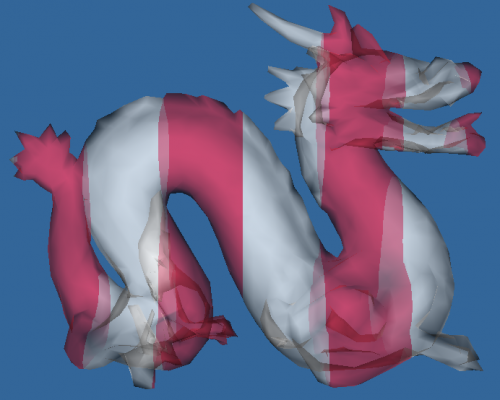### 顺序无关半透明：Weighted Blended

所有基于排序的 OIT 算法实际上都需要大量的计算资源，不管是基于图元的排序还是基于像素的排序。

## Blend 中的 OVER 操作

Blend 计算的基本思想是:
C_f=C_1+(1-\alpha_1)C_0　　这里，与上一篇博客 顺序无关半透明：Depth Peeling 中的定义一样，C_0 表示 premultiplied-alpha color，预先乘上了 \alpha，即 color.rgb * color.a。
那么，当有 n 个半透明物体覆盖在背景上时，有
C_f=[C_n+(1-\alpha_n) {\cdot}{\cdot}{\cdot}[C_2+(1-\alpha_2)[C_1+(1-\alpha_1)C_0]]{\cdot}{\cdot}{\cdot}]　　所以，背景 C_0 的贡献为：
C_0\prod_{i=1}^n(1-\alpha_i)　　同理，C_i 的贡献为：
C_i\prod_{j=i+1}^n(1-\alpha_j)　　由于上述累乘刨除掉 C_i 后实际上是顺序无关的，所以如何将 C_i 通过解析式估计出来，也就成了问题的关键。最终的 Weighted Blended 将上述式子融合为一个估计的解析式，它有一定偏差，但是在非极端情况下已经非常够用。

## Weighted Blended OIT

在不使用特殊缓冲区和排序的情况下，可以用 Weighted Blended OIT实现依赖现有数据和BlendFunction的OIT。 下面的介绍中对推导过程进行了省略，因为里边有大量的经验公式。

### Meshkin的方法

C_f=(\sum_{i=1}^nC_i)+C_0(1-\sum_{i-1}^n\alpha_i)　　这里，每次执行像素着色器之后，将 premultiplied 得到的 C_i 以及 \alpha_i 各自累加起来，最后通过单独的着色器将两者合并即可。
这时，当 \alpha 的值很小并且颜色相似时可以得到较好的效果（类似于排序OIT），但随着 \alpha 的值增加，差距会越来越明显。

### Bavoil和Myer的方法

C_f={\sum_{i=1}^nC_i\over \sum_{i=1}^n\alpha_i}\cdot(1-[1-{1\over n}\sum_{i=1}^n\alpha_i]^n)+C_0[1-{1\over n}\sum_{i=1}^n\alpha_i]^n　　新的方法在 Meshkin 方法中引入了“加权平均”算子。[1-{1\over n}\sum_{i=1}^n\alpha_i]^n 被称为总透明度。根据计算的总透明度，然后通过 C_i\alpha_i 的加权估计最终的颜色。

### 新的 Blended OIT

C_f={\sum_{i=1}^nC_i\over \sum_{i=1}^n\alpha_i}(1-\prod_{i=1}^n(1-\alpha_i))+C_0\prod_{i=1}^n(1-\alpha_i)　　在其它方法中，\alpha=0 以及 C_i=0 的情况下才有助于估计真实的半透明颜色。
新的方法中，\prod_{i=1}^n(1-\alpha_i) 被估计为总透明度。对于半透明颜色，使用上述方法中的 \alpha_i 的加权平均值计算，计算最终的半透明颜色。

### 增加深度权重

C_f={\sum_{i=1}^nC_i\cdot w(z_i, \alpha_i)\over \sum_{i=1}^n\alpha_i\cdot w(z_i, \alpha_i)}(1-\prod_{i=1}^n(1-\alpha_i))+C_0\prod_{i=1}^n(1-\alpha_i)　　由于上一个 Blend 公式中获得的半透明物体颜色是简单 \alpha 的加权平均值。所以不管 \alpha 权值大的像素上面还有多少层半透明像素，它都会是非常明显的一部分。
所以，这里不仅使用简单的 \alpha 加权平均，将深度也作为权重标准之一，即相同 \alpha 的片段，距离相机的距离越近，则权重越大。计算出的贡献增大到视点越近。
权重函数的选取要求单调递减，这里无法使用解析的公式推导，归根结底，只是经验公式罢了。以下四种权重函数可以提供参考：
\begin{aligned} w(z,\alpha)&=\alpha\cdot max[10^{-2},min[3\times10^{3},{10\over 10^{-5}+(\vert z\vert /5)^2+(\vert z\vert /200)^6}]]\\ w(z,\alpha)&=\alpha\cdot max[10^{-2},min[3\times10^{3},{10\over 10^{-5}+(\vert z\vert /10)^3+(\vert z\vert /200)^6}]]\\ w(z,\alpha)&=\alpha\cdot max[10^{-2},min[3\times10^{3},{10\over 10^{-5}+(\vert z\vert /200)^4}]]\\ w(z,\alpha)&=\alpha\cdot max[10^{-2},3\times10^3\cdot(1-d(z))^3] \end{aligned}　　上述第四个式子中的 z 表示 OpenGL 中 gl_FragCoord.z，在相机空间为负值。有
d(z)={(z_{near}z_{far})/z-z_{far}\over z_{near}-z_{far}}

## 分析

Weighted Blended OIT 是一种有效的手段，因为它可以节省半透明排序的时间，并且可以避免排序带来的 GPU 负载不均衡的问题。在笔记本 950M 中可以跑 300+ fps，相比 Depth Peeling 的 100 fps 要太多了。
当然，估计肯定会带来误差。Weighted Blended OIT 还是有加权平均的传统矛盾，当 \alpha 值比较大偏向于不透明的时候，误差会越来越大，并不能完全达到基于排序 OIT 的效果。

## 结果Tags :

### About the Author## 2 thoughts on “顺序无关半透明：Weighted Blended”

•Get Clobetasol [url=http://cialonlineno.com]buy generic cialis online[/url] Prix Viagra Boite De 8 Online Keflex No Rx Needed Comprar Lamisil Pastillas Que Es Cialis O Viagra [url=http://drugsed.com][/url] Viagra Frau Bericht Buy Amoxicillin Online In The Uk [url=http://rxbill6.com]compare viagra to cialis and levitra[/url] Keflex For Bronchitis Viagra Ohne Rezept Review Amoxicillin And Vitamin Interactions Kamagra Online Prescription [url=http://gaprap.com]viagra online[/url] Celexa Online Pharmacy Cheap Levitra Canada [url=http://cialiprice.com]cialis generic[/url] Achat Cialis Pharmacie Cialis Tadalafil 100 Dogs Cephalexin 250 Mg Canada [url=http://arealot.com]vytorin[/url] Discount Cheap Amoxicilina Online Buy Generic Propecia

•It’s perfect time to make some plans for the long run and it’s time to be happy.
I have read this submit and if I could I wish to suggest you few fascinating things or suggestions.
Perhaps you could write next articles referring to this
article. I wish to learn more things approximately it!